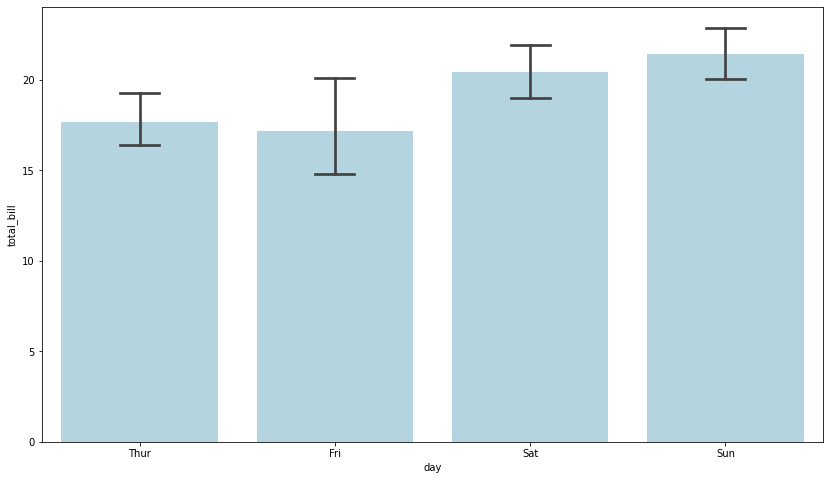If you are using aggregated values in a barplot, error bars will give you a general idea of how precise a measurement is. This example shows how to add error bars into your chart.

By default, the `barplot()` function draws error bars in the plot with 95% confidence interval. You can remove error bars by passing `ci=None` argument. `ci` parameter controls the size of confidence intervals to draw around estimated values (Note that if you want to draw the standard deviation of the observations, you should pass `ci="sd"`).

Additionally, you can change the width of the caps on error bars with the `capsize` parameter.

``````# import libraries
import seaborn as sns
import numpy as np
import matplotlib.pyplot as plt

# Set the figure size
plt.figure(figsize=(14, 8))

# plot a bar chart
ax = sns.barplot(x="day", y="total_bill", data=tips, estimator=np.mean, ci=85, capsize=.2, color='lightblue')``````## Contact & Edit

👋 This document is a work by Yan Holtz. You can contribute on github, send me a feedback on twitter or subscribe to the newsletter to know when new examples are published! 🔥